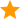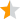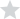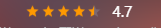# Detailed explanation of the example of star rating effect realized by Vue

Time：2021-1-10

We separate the star rating into a star component and separate it from the parent component (the score value is passed in)

``````
<div>
<Star :score="poiInfo.wm_poi_score"></Star>
</div>``````

initial Star.vue

``````
<template>
<div>
<div>
<span></span>
</div>
<span>{{ score }}</span>
</div>
</template>
<script>
export default {
name: 'Star',
props: {
score: Number
}
}
</script>``````

First of all, we cycle the star image with the span tag of star item. There are three star images: full star, half star and empty starThe following only lists the key parts of CSS, and distinguishes images by adding classes

``````
.star-item.on {
background-image: url(./img/[email protected]);
}
.star-item.half {
background-image: url(./img/[email protected]);
}
.star-item.off {
background-image: url(./img/[email protected]);
}``````

Next, modify it Star.vue Code for

``````
<div>
<span

v-for="(itemClass, index) in itemClasses"
:key="index"
:class="itemClass"
>
</span>
</div>``````

The itemclasses value is obtained by calculating attributes

Return an array with length of 5 through computed (display 5 stars)

The value of the array is the class name corresponding to the different stars taken by the above CSS, and then by binding the class added by each loop, the star can be traversed.

For example:

4.7 points, the corresponding array is [‘on ‘,’on’,’on ‘,’on’,’half ‘]

The corresponding array of 3.4 points is [‘on ‘,’on’,’on ‘,’half’,’half ‘]

JS code:

``````//Star length
const LENGTH = 5

//The state of the stars
const CLS_ON = 'on'
const CLS_HALF = 'half'
const CLS_OFF = 'off'

export default {
name: 'Star',
props: {
score: Number
},
computed: {
itemClasses () {
let result = []

let score = Math.floor(this.score * 2) / 2

//Half star
let hasDecimal = score % 1 !== 0

//Whole star (round down to get the whole star)
let integer = Math.floor(score)

//Traversing all stars
for(let i = 0; i < integer; i++){
result.push(CLS_ON)
}

//Processing half stars
if(hasDecimal){
result.push(CLS_HALF)
}

//Make up
while( result.length  < length) {// if there are not enough five stars here, we will try to find a space star
result.push(CLS_OFF)
}

return result
}
}
}``````

Finally, itemclasses returns an array with a length of 5

`let score = Math.floor(this.score * 2) / 2`

Half Star Division: only when the first decimal place is greater than or equal to 5 can be regarded as half star, otherwise it is empty star. The purpose of this calculation is to determine whether it is a half star by taking the remainder of 1 after the decimal part > = 0.5. It’s a bit confusing at the beginning. Just try a few more.

For example, there is no half star at 4.3 and half star at 4.5

result:

For example, if the value passed in is 4.7, thesummary

The above is the detailed explanation of Vue star rating effect introduced by Xiaobian. I hope it can help you. If you have any questions, please leave me a message and Xiaobian will reply you in time. Thank you very much for your support to developer!